# GSEB Solutions Class 12 Maths Chapter 9 Differential Equations Miscellaneous Exercise

Gujarat Board GSEB Textbook Solutions Class 12 Maths Chapter 9 Differential Equations Miscellaneous Exercise Textbook Questions and Answers.

## Gujarat Board Textbook Solutions Class 12 Maths Chapter 9 Differential Equations Miscellaneous Exercise

Question 1.
For each of the differential equations given below, indicate its order and degree (if defined):
(i) $$\frac{d^{2} y}{d x^{2}}$$ + 5x($$\frac{dy}{dx}$$)2 – 6y = log x
(ii) ($$\frac{dy}{dx}$$)3 – 4($$\frac{dy}{dx}$$)2 + 7y = sin x
(iii) $$\frac{d^{4} y}{d x^{4}}$$ – sin ($$\frac{d^{3} y}{d x^{3}}$$) = 0
Solution:
(i) Order = 2, Degree = 1
(ii) Order 1, Degree = 3
(iii) Order 4, Degree not defined.Question 2.
For each of the questions given below, verify that the given function (implicit of explicit) is a solution of the corresponding differential equation:
(i) y = a ex + b e-x + x2 : x $$\frac{d^{2} y}{d x^{2}}$$ + 2$$\frac{dy}{dx}$$ – xy + x2 – 2 = 0
(ii) y = ex(a cos x + b sin x) : $$\frac{d^{2} y}{d x^{2}}$$ – 2$$\frac{dy}{dx}$$ + 2y = 0
(iii) y = x sin 3x : $$\frac{d^{2} y}{d x^{2}}$$ + 9y – 6 cos 3x = 0
(iv) x2 = 2y2 log y : (x2 + y2)$$\frac{dy}{dx}$$ – xy = 0
Solution:
(i) y = a ex + b e-x + x2
∴ $$\frac{dy}{dx}$$ = a ex – be-x + 2x
and $$\frac{d^{2} y}{d x^{2}}$$ = a ex + b e-x + 2
Now, x $$\frac{d^{2} y}{d x^{2}}$$ + 2$$\frac{dy}{dx}$$ – xy + x2 – 2
= x(a ex + b e-x + 2) + 2(a ex – be-x + 2x) – x(a ex + b e-x + x2) + x2 – 2
= 2x + 2(a ex – b e-x) + 6x – x3 + x2 – 2 = 0
= 2(a ex – b e-x) – x3 + x2 + 6x – 2 ≠ 0.
Hence, y = a ex + b e-x + x2 is not the solution of the differential equation $$\frac{d^{2} y}{d x^{2}}$$

(ii) y = ex (a cos x + b sin x)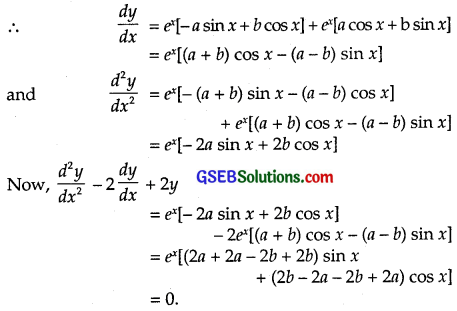Hence, y = ex(a cos x + b sin x) is the solution of the differential equation $$\frac{d^{2} y}{d x^{2}}$$ – 2$$\frac{dy}{dx}$$ + 2y = 0

(iii) y = x sin 3x, So $$\frac{dy}{dx}$$ = sin 3x + 3x cos 3x
and $$\frac{d^{2} y}{d x^{2}}$$ = 3 cos 3x + 3[cos 3x – 3x sin 3x]
= 6 cos 3x – 9x sin 3x = 6 cos 3x – 9y
⇒ $$\frac{d^{2} y}{d x^{2}}$$ + 9y – 6 cos 3x = 0
Hence, y = x sin 3x is the solution of $$\frac{d^{2} y}{d x^{2}}$$ + 9y – 6 cos 3x = 0.

(iv) x2 = 2y2 log y ………………… (1)
Differentiating w.r.t.x, we get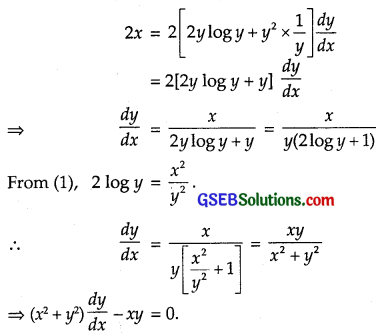Hence, x2 = 2y2 log y is the solution of differential equation (x2 + y2) $$\frac{dy}{dx}$$ – xy = 0.Question 3.
Form the differential equation representing the family of curves given by (x – a)2 + 2y2 = a2, where a is an arbitrary constant.
Solution:
The equation of the curve is (x – a)2 + 2y2 = a2
or x2 – 2ax + a2 + 2y2 = a2
i.e; x2 + 2y2 – 2ax = 0 ……………… (1)
Differentiating w.r.t x, we get
2x + 4y $$\frac{dy}{dx}$$ – 2a = 0
Multiply it with x to obtain
2x2 + 4xy $$\frac{dy}{dx}$$ – 2ax = 0 …………………. (2)
substracting (1) from (2),
x2 – 2y2 + 4xy $$\frac{dy}{dx}$$ = 0.
∴ Required differential equation is
4xy $$\frac{dy}{dx}$$ + x2 – 2y2 = 0
or $$\frac{dy}{dx}$$ = $$\frac{2 y^{2}-x^{2}}{4 x y}$$.

Question 4.
Prove that x2 – y2 = c(x2 + y2)2
is the general solution of differential equation (x2 – 3xy2) dx = (y3 – 3x2y) dy, when c is the parameter.
Solution:
The differential equation is (x3 – 3xy2)dx = (y3 – 3x2y)dy
∴ $$\frac{dy}{dx}$$ = $$\frac{x^{3}-3 x y^{2}}{y^{3}-3 x^{2} y}$$
which is a homogeneous equation.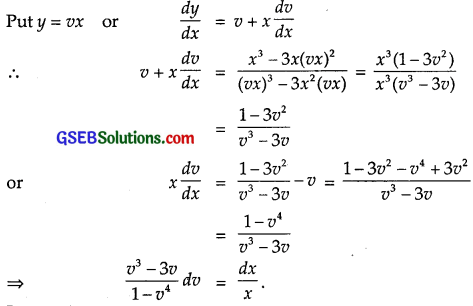Integrating, we getPut 1 – v4 = t, so that – 4v3dv = dt.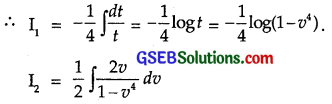Put v2 = t, so that 2v dv = dt.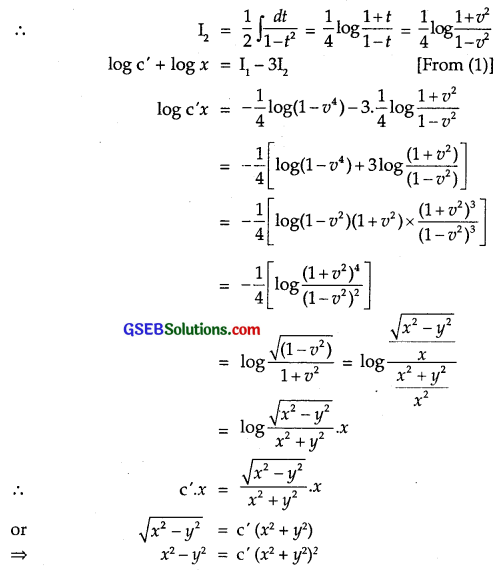Putting c’ = c, we get
x2 – y2 = c(x2 + y2)2
Hence, x2 – y2
= c(x2 + y2)2 is the solution of the differential equation (x3 – 3xy2)dx
= (y3 – 3x2y) dy, where c is a parameter.Question 5.
Form the differential equation of the family of circles in the first quadrant which touch the coordinate axes.
Solution:
Family of circles which touch the coordinates axes in first quadrant is
(x – a)2 + (y – a)2 = a2 …………………. (1)where a is the radius of the circle.
Differentiating w.r.t. x we get,
2(x – a) + 2(y – a)$$\frac{dy}{dx}$$ = 0
Put $$\frac{dy}{dx}$$ = p.
∴ (x – a) + (y – a)p = 0 or x – a + py – pa = 0
x + py – a(1 + p) = 0 ∴ a = $$\frac{x+py}{1+p}$$.
Putting value of a in (1), we get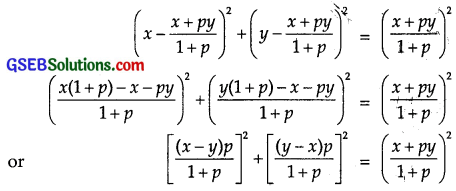Multiplying both sides by (1 + p)2,
(x – y)2p2 + (x – y)2 = (x + py)2
or (x – y)2(1 + p2) = (x + py)2
⇒ (x – y)2[1 + ($$\frac{dy}{dx}$$)2] = [x + y$$\frac{dy}{dx}$$]2
This is the required diff.equation.

Question 6.
Find the general solution of the differential equation $$\frac{dy}{dx}$$ + $$\sqrt{\frac{1-y^{2}}{1-x^{2}}}$$ = 0.
Solution:
The differential equation is $$\frac{dy}{dx}$$ + $$\sqrt{\frac{1-y^{2}}{1-x^{2}}}$$ = 0.
or $$\frac{d y}{\sqrt{1-y^{2}}}$$ + $$\frac{d x}{\sqrt{1-y^{2}}}$$ = 0.
Integrating, we get
∫ $$\frac{d y}{\sqrt{1-y^{2}}}$$ + ∫ $$\frac{d x}{\sqrt{1-y^{2}}}$$ = C
or sin-1y + sin-1x = C.
This is the required solution.Question 7.
Show that the general solution of the differential equation
$$\frac{dy}{dx}$$ + $$\frac{y^{2}+y+1}{x^{2}+x+1}$$ = 0 is given by x + y + 1 = A(1 – x – y – 2xy), where A is parameter.
Solution:
The differential equation is
$$\frac{dy}{dx}$$ + $$\frac{y^{2}+y+1}{x^{2}+x+1}$$ = 0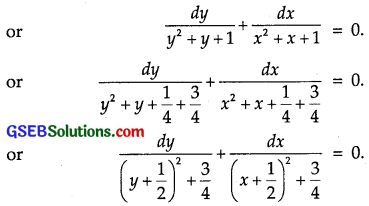Integrating we have,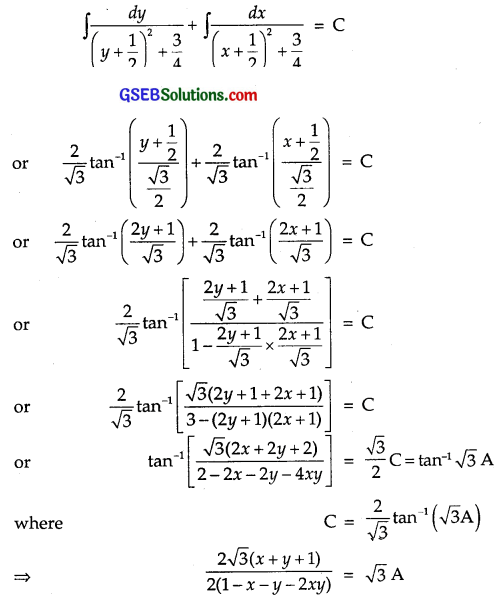∴ Required solution is x + y + 1 = A(1 – x – y – 2xy).

Question 8.
Find the equation of the curve passing through the point (0, $$\frac{π}{4}$$), whose differential equation is sin x cos y dx + cos x sin y dy = 0.
Solution:
The given differential equation is
sin x cos y dx + cos x sin y dy = 0
Dividing by cos x cos y,
$$\frac{sinx}{cosx}$$dx + $$\frac{siny}{cosy}$$dy = 0
Integrating, we get
∫tan x dx + ∫tan y dy = log C
log sec x + log sec y = log C
or log sec x sec y = log C
or sec x sec y = C.
Curve passes through the point (0, $$\frac{π}{4}$$)
∴ sec 0 sec $$\frac{π}{4}$$ = C ⇒ C = $$\sqrt{2}$$.
Hence, the required equation of the curve is sec x sec y = $$\sqrt{2}$$.Question 9.
Find the particular solution of the differential equation (1 + e2x)dy + (1 + y2)ex dx = 0, given that y = 1, when x = 0.
Solution:
The differential equation is
(1 + e2x)dy + (1 + y2)ex dx = 0
Dividing by (1 + e2x)(1 + y2), we get
$$\frac{d y}{1+y^{2}}$$ + $$\frac{e^{x} d x}{1+e^{2 x}}$$ = 0.
Integrating, we get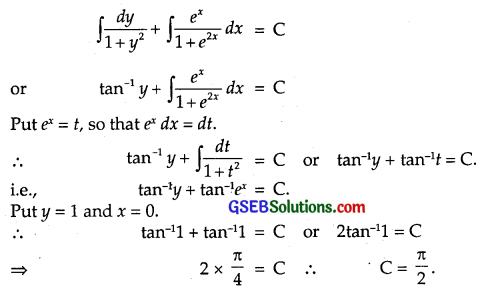∴ The particular solution of the given differential equation is
tan-1y + tan-1ex = $$\frac{π}{2}$$.

Question 10.
Solve the differential equation y ex/y dx = (x ex/y + y2) dy.
Solution:
The differential equation is y ey/x $$\frac{dx}{dy}$$ = x ex/y + y2.Question 11.
Find a particular solution of the differential equation (x – y)(dx + dy) = dx – dy, given that y = – 1, when x = 1.
Solution:
The differential equation is (x – y) (dx + dy) = dx – dy.
or (x – y + 1)$$\frac{dy}{dx}$$ + x – y – 1 = 0.Integrating we get,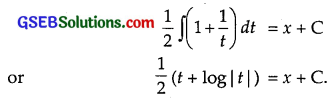Putting t = x – y, we get
$$\frac{1}{2}$$[(x – y) + log |x – y|] = x + C
When x = 0, y = – 1.
$$\frac{1}{2}$$(1 + 0) = 0 + C C = $$\frac{1}{2}$$.
∴ Solution is
$$\frac{1}{2}$$[(x – y) + log|x – y|] = x + $$\frac{1}{2}$$
or x – y + log |x – y| = 2x + 1
or y = – (x + 1) + log |x – y|
or log|x – y| = x + y + 1.Question 12.
Solve the differential equation ($$\frac{e^{-2 \sqrt{x}}}{\sqrt{x}}-\frac{y}{\sqrt{x}}$$) $$\frac{dx}{dy}$$ = 1.
Solution:
The differential equation is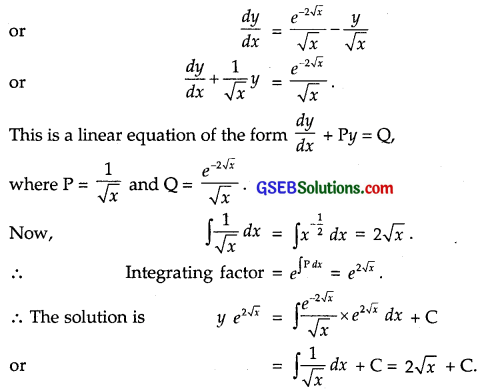∴ Required solution is $$y e^{2 \sqrt{x}}$$ = 2$$\sqrt{x}$$ + C.

Question 13.
Find the particular solution of the differential equation $$\frac{dy}{dx}$$ + y cot x = 4x cosec x (x ≠ 0); given that y = 0,
when x = $$\frac{π}{2}$$.
Solution:
The differential equation is $$\frac{dy}{dx}$$ + y cot x = 4x cosec x.
This is a linear equation of the form $$\frac{dy}{dx}$$ + Py = Q
where P = cot x and Q = 4x cosec x.
∴ ∫Pdx = ∫cot x dx = log sin x.
or I.F. = e∫Pdx = elogsinx = sin x.
∴ The solution is y sin x = ∫4x cosec x sin x dx + C
= ∫4x dx + C = 2x2 + C
i.e; y sin x = 2x2 + C
Putting y = 0, x = $$\frac{π}{2}$$, we get
0 = 2 × $$\frac{\pi^{2}}{4}$$ + C
⇒ C = – $$\frac{\pi^{2}}{2}$$.
∴ Required particular solution is
y sin x = 2x2 – $$\frac{\pi^{2}}{2}$$, (sin x ≠ 0).Question 14.
Find a particular solution of the differential equation (x + 1) $$\frac{dy}{dx}$$ = 2e-y – 1; given that y = 0, when x = 0.
Solution:
The differential equation
(x + 1) $$\frac{dy}{dx}$$ = 2e-y – 1.
Varaibles are separable.Integrating, we getPut 2 – ey = t, so that – ey dy = dt.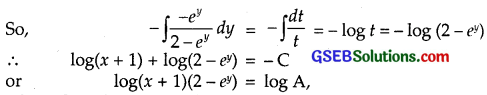∴ log(x + 1) + log(2 – ey) = – C
or log(x + 1)(2 – ey) = A,
Where C = – log A.
∴ (x + 1)(2 – ey) = A.
Putting x = 0 and y = 0, we get
2 – 1 = A = 1.
∴ Required particular solution is (x + 1)(2 – ey) = 1.
or 2 – ey = $$\frac{1}{x+1}$$.
or ey = 2 – $$\frac{1}{x+1}$$ = $$\frac{2x+1}{x+1}$$.
∴ y = log |$$\frac{2x+1}{x+1}$$|, x ≠ – 1.

Question 15.
The population of a village increases at the rate proportional to the number of its inhabitatants present at any time.
If the population of the village was 20000 in 1999 and 250000 in the year 2004, what will be the population of the village in 2009?
Solution:
Let y be the population at any instant t.
Now, population increases at a rate ∝ Number of inhabitants.
∴ $$\frac{dy}{dt}$$ ∝ y or $$\frac{dy}{dt}$$ = ky,
where k is the constant of proportionality.
∴ $$\frac{dy}{y}$$ = k dt
Integrating we get,
∫$$\frac{dy}{y}$$ = ∫k dt + C
or log y = kt + C …………….. (1)
In 1999 at t = 0, population y = 20000
∴ log 20000 = C.
Putting value of C in (1), we get
log y = kt + log 20000
or log y – log 20000 = kt
or log $$\frac{y}{20000}$$ = kt
In 2004, at t = 5, y = 25000.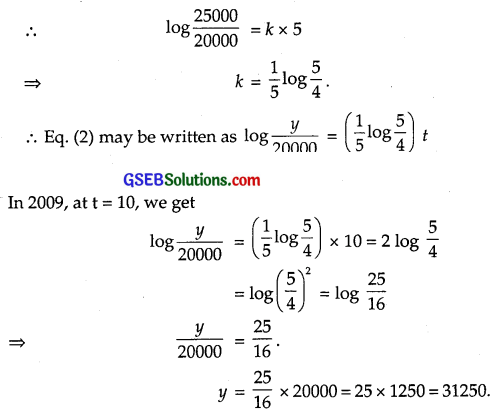Choose the correct answers in the following questions 16 to 18:

Question 16.
The general solution of the differential equation $$\frac{ydx-xdy}{y}$$ = 0 is
(A) xy = C
(B) x = Cy2
(C) y = Cx
(D) y = Cx2
Solution:
The differential equation is $$\frac{ydx-xdy}{y}$$ = 0
or dx – $$\frac{x}{y}$$ dy = 0 or $$\frac{dx}{x}$$ – $$\frac{dy}{y}$$ = 0
Integrating, we get
∫$$\frac{dx}{x}$$ – ∫$$\frac{dy}{y}$$ = C’
⇒ log x – log y = C’ or $$\frac{x}{y}$$ = C’
Putting C’ = $$\frac{1}{C}$$, we get
$$\frac{x}{y}$$ = $$\frac{1}{C}$$.
So, y = Cx is the required solution.
∴ Part (C) is the correct answer.

Question 17.
The general solution of differential equation of the type $$\frac{dy}{dx}$$ + P1y = Q1 is
(A) y $$e^{\int P_{1} d y}$$ = ∫(Q1$$e^{\int P_{1} d y}$$)dy + C
(B) y e$$e^{\int P_{1} d y}$$ = ∫(Q1$$e^{\int P_{1} d x}$$)dx + C
(C) x e$$e^{\int P_{1} d y}$$) = ∫(Q1$$e^{\int P_{1} d x}$$)dy + C
(D) x e$$e^{\int P_{1} d y}$$) = ∫(Q1$$e^{\int P_{1} d x}$$)dx + C
Solution:
The linear differential equation is $$\frac{dx}{dy}$$ + P1x = Q1,
where P1 and Q1 are the functions of y.
∴ I.F. = $$e^{\int \mathrm{P}_{1} d y}$$
Hence, the solution is
x.$$e^{\int \mathrm{P}_{1} d y}$$ = ∫(Q1 × $$e^{\int \mathrm{P}_{1} d y}$$)dy + C
∴ Part (C) is the correct answer.Question 18.
The general solution of the differential equation exdy + (yex + 2x)dx = 0 is
(A) xey + x2 = C
(B) xey + y2 = C
(C) yex + x2 = C
(D) yey + x2 = C
Solution:
The differential equation is ex dy + (y ex + 2x) dx = 0
or ex $$\frac{dy}{dx}$$ + exy = – 2x
or $$\frac{dy}{dx}$$ + 1.y = – 2xe-x
I.F. = e∫dx = ex
∴ Solution is y ex = ∫(- 2x)e-x × ex dx + C
= – ∫2x dx + C = – x2 + C
⇒ y ex + x2 = C
∴ Part (C) is the correct answer.